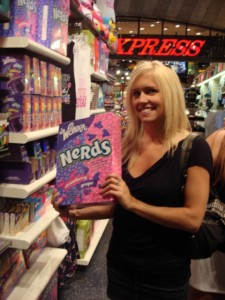### Review: Calling Functions

So far in this course, we have called functions to perform calculations or create images.

Examples:

(* 2 3) multiplies 2 × 3

(square 40 "solid" "red") displays a red square

### Functions in Algebra

We use function notation to define functions.

$$f(x)=x+3$$

To evaluate the above function when #&x=2#&, we write

$$f(2) = 2+3$$

#&f(2)#& means replace #&x#& in the expression with 2.

### Functions in Programs

We use the keyword define to define a new function. For the above example, we would write:

(define (f x) (+ x 3))

To find the value of the function when x is 2, use the interactions window to call the function.

(f 2)

#### Important!

• Define new functions in the Definitions window
• Click Run after writing a definition
• Call the function using the Interactions window

#### Example: Calculating Total Cost

 Suppose the price of a giant box of Nerds is \$10.25. How much does it cost to buy four boxes? To find the total cost of items, we multiply price × quantity. Here is the definition of a function to do that: (define (total-cost price quantity) (* price quantity)) The name of function is total-cost. The variables price and quantity are called the parameters of the function. When we call total-price, the parameters are bound with the actual values.For example, if we type (total-cost 10.25 4), then price is 10.25, and quantity is 4.

#### Binding Arguments to Parameters#### Defining Functions to Simplify Life

Suppose we want to create rectangles that are always solid and red. We can define the following function:

(define (red-rectangle width height) (rectangle width height "solid" "red"))

Then we can create solid rectangles by typing

(red-rectangle 40 20)

#### Functions to Perform Calculations

The Pythagorean Theorem shows the relationship among sides of a right triangle: $$a^2+b^2=c^2$$ We can use this theorem to find the length of the hypotenuse: $$c= \sqrt {a^2+b^2}$$

In a program, we might label the legs of the triangle leg-1 and leg-2 instead of #&a#& and #&b#&. Here is the function to find the hypotenuse:

(define (hypotenuse leg-1 leg-2) (sqrt (+ (* leg-1 leg-1) (* leg-2 leg-2)))

### Program Exercise 3.2 - Defining Functions

Write a function definition for each of the following calculations. Test your definitions using the interactions window. If you need help, press the Hint button. If you're really stuck, press Show Me.

1. The formula for area of a rectangle is length × width.

Write a define statement in the Definitions window. It starts like this: (define (area length width) ...)

(define (area length width) (* length width))

2. The formula for distance traveled is rate × time.

This definition is just like the last one, but the names are different.

(define (distance rate time) (* rate time))

3. Write a function to find the area of a triangle, given its base and height.

The area of a triangle is base × height / 2.

(define (area-triangle base height) (/ (* base height) 2))

4. The area of a trapezoid is #&A=\frac {(b_1+b_2)h}{2}#&.

Use these variable names: trap-area, base_1, base_2, height

(define (trap-area base_1 base_2 height) (/ (* (+ base_1 base_2) height) 2))

5. Write a function to find the volume of a right rectangular pyramid, given its base length, base width, and height.

Area of a pyramid

(define (pyramid-volume base_length base_width height) (/ (* (* base_length base_width) height) 3))

6. Write a function to find slope of a line passing through two points.

#&m= \frac {y_2-y_1} {x_2-x_2}#&

(define (slope y_2 y_1 x_2 x_1) (/ (- y_2 y_1) (- x_2 x_1) ))

### Challenge Exercises

7. Write a function that finds (produces) the distance between two points #&(x_1, y_1)#& and #&(x_2, y_2)#&.

Use the distance formula, which is derived from the Pythagorean Theorem. Test this function using these points: (1,1) and 5,4).

8. Write a function that finds (produces) the perimeter of a triangle, given three points #&(x_1, y_1)#&, #&(x_2, y_2)#&, and #&(x_3, y_3)#&.

This function should use the function you wrote for exercise 7.

9. Write a function that uses Heron's Formula to find the area of a triangle define by three points. #&(x_1, y_1)#&, #&(x_2, y_2)#&, and #&(x_3, y_3)#&.
Heron's Formula: #&A=\sqrt {p(p-a)(p-b)(p-c)}#&, where #&p#& is half the perimeter of the triangle, and #&a,b,c#& are the side lengths.

It is possible to write this function using the function defined in exercise 8. However, this new function will be very long an tedious. There are better ways of solving this problem, which you will learn in a later lesson.

### Program Exercise 3.3 - Finding Errors

The following function definitions contain syntax errors. For each function, do the following:

1. Use Dr. Racket's definitions window to find and correct the syntax error.
2. Test the function using the interactions window.
3. Write the corrected function definition and test results in your workbook.

1.
;; This function should create a red square
(define (red-square side-length) (square sideLength "solid" "red"))

2.
;; This function finds the perimeter of a square
(define square-perimeter side-length) (* side-length 4))

3.
;; This function finds the perimeter of an equilateral triangle
(define (equilateral-triangle-perimeter side-length) (side-length * 3))

4.
;; This function finds the area of a triangle
(define (triangle area base height) (/ (* base height) 2)

5.
;; THis function converts feet to inches
(define feet->inches(feet) (* feet 12))

6.
;; This function calculates density
define (density mass volume) (/ mass volume)

7.
;; This function finds the product of three numbers
(define (product-3 number1 number2 number3) (number1 * number2 * number3)))

8.
;; This function finds the sum of three numbers
(define (sum-3 number1 number2 number3) number1 + (+ number2 number3)))

9.
;; This function finds the average of three numbers
(define (average-3 number number number) (/ (sum-3 number number number) 3))

Computer Programming Unit 3.2 - Defining Functions